# One theta to rule them all: Test Individual Differences

#### 23 augustus, 2017

Educational and psychological testing is all about individual differences. Using an instrument, we try our best to place an individual with respect to others depending on their level of extroversion, depression, or mastery of English.

What if there are no individual differences at all? We would find ourselves in the unenviable position of having no Snark to hunt for, no ghosts to bust. Dexter includes a function, individual_differences, to check whether the response data are consistent with the hypothesis of no individual differences in true ability.

Here is a simple function to simulate a matrix of response data from the Rasch model, in the long shape expected by dexter. We use it to generate responses to 20 items with uniformly distributed difficulties from 2000 persons having all the same true ability of 0.5:

sim_Rasch = function(theta, delta) {
n = length(theta)
m = length(delta)
data.frame(
person_id = rep(paste0('p',1:n), m),
item_id = rep(paste0('i',1:m), each=n),
item_score = as.integer(rlogis(n*m, outer(theta, delta, "-")) > 0)
)
}

simulated = sim_Rasch(rep(0.5, 2000), runif(20, -2, 2))

Computing the sum scores and examining their distribution, we find nothing conspicuous:

ss= simulated %>%
group_by(person_id) %>%
summarise(sumscore=sum(item_score))
par(mfrow=c(1,2))
hist(ss$sumscore) plot(ecdf(ss$sumscore))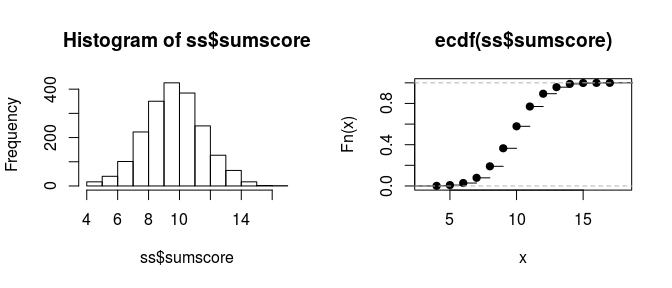mm = fit_inter(simulated)

We can also examine the various item-total regressions produced by function fit_inter. For example, here are the plots for the first two items:

mm = fit_inter(simulated)
par(mfrow=c(1,1))
plot(mm, show.observed = TRUE,
items = c('i1','i2'),
nr=1, nc=2)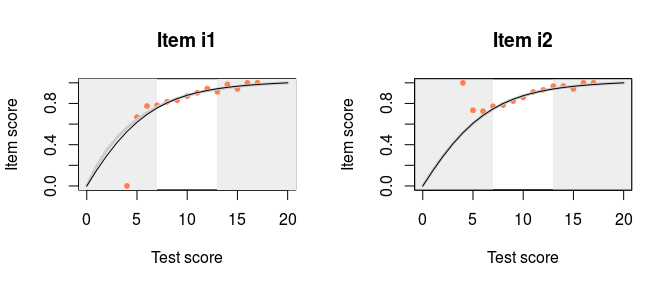The curtains that eliminate the 5% smallest and 5% largest sum scores are drawn somewhat narrow but, apart from that, all regressions look nice. It appears that, by just looking at the response data, we are not in a very good position to judge whether there are any true individual differences in ability. To help with that, dexter offers a function, individual_differences:

dd = individual_differences(simulated)
## =
plot(dd)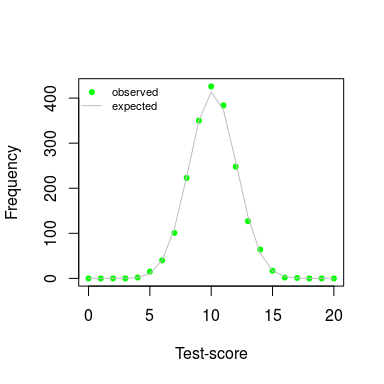The gray line shows the predicted frequency of each sum score under the hypothesis of no true individual differences. The green dots show the observed frequencies. We now see that our observed data is compatible with the null hypothesis.

The print function for the test shows a chi-squared test for the null hypothesis. This uses R’s option to simulate the p-value, which explains why the degrees of freedom are missing:

print(dd)
##  "Chi-Square Test for the hypothesis that all respondents have the same ability:"
##
##  Chi-squared test for given probabilities with simulated p-value
##  (based on 2000 replicates)
##
## data:  observed
## X-squared = 7.1267, df = NA, p-value = 0.938

Thus, we find a p-value of 0.94 for the hypothesis that there are no individual differences.

What about real data? Dexter comes with a well-known example preinstalled, the verbal aggression data (Vansteelandt 2000) analysed in great detail in (Paul De Boeck 2004) and many others. 243 females and 73 males have assessed on a 3-point scale (‘yes’, ‘perhaps’, or ‘no’) how likely they are to become verbally aggressive in four different frustrating situations

db2 = start_new_project(verbAggrRules, "verbAggression.db")
add_booklet(db2, verbAggrData, "data")
## $items ##  "S1DoCurse" "S1DoScold" "S1DoShout" "S1WantCurse" "S1WantScold" ##  "S1WantShout" "S2DoCurse" "S2DoScold" "S2DoShout" "S2WantCurse" ##  "S2WantScold" "S2WantShout" "S3DoCurse" "S3DoScold" "S3DoShout" ##  "S3WantCurse" "S3WantScold" "S3WantShout" "S4DoCurse" "S4DoScold" ##  "S4DoShout" "S4WantCurse" "S4WantScold" "S4WantShout" ## ##$covariates
## character(0)
##
## $columns_ignored ##  "Gender" ## ##$auto_add_unknown_rules
##  TRUE
##
## $zero_rules_added ##  item_id response ## <0 rows> (or 0-length row.names) dd = individual_differences(db2, booklet_id=="data") ## == plot(dd)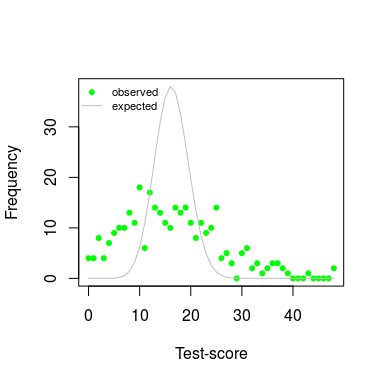This is quite different now, and the chi-squared test is highly significant, with a p-value of 510^{-4}. ## Taking uncertainty in item parameters into account In a Bayesian framework, we are not forced to assume that item parameters are fixed and known quantities. We can take samples from their posterior distributions to take into account the fact that we are not dealing with true values. The current version of dexter does not include a user-level function to do that, but we can easily tinker with some of its internal functions. Function theta_score_distribution estimates ability from a score distribution, and function pscore produces the expected score distribution given an ability and item parameters. First, we run a Bayesian calibration and store the results for convenience. We will use the simulated data from the first example. f_b = fit_enorm(simulated, method="Bayes") b = colMeans(f_b$est$b) a = f_b$est$a first = f_b$inputs$ssI$first
last = f_b$inputs$ssI$last scoretab = f_b$inputs$stb$N

We then produce a plot of observed and estimated frequencies.

plot(0:sum(a[last]), scoretab, col="green", pch=19,
xlab="Test-score", ylab="Frequency", cex=0.7)

theta.est = dexter:::theta_score_distribution(b,a,first,last,scoretab)
score.est = dexter:::pscore(theta.est,b,a,first,last)

for (i in 1:20)
{
indx=sample(1:500,1)
b=f_b$est$b[indx,]
theta.est=dexter:::theta_score_distribution(b,a,first,last,scoretab)
score.est=dexter:::pscore(theta.est,b,a,first,last)
lines(0:sum(a[last]),score.est*2000,col="gray",pch=19,cex=0.7)
}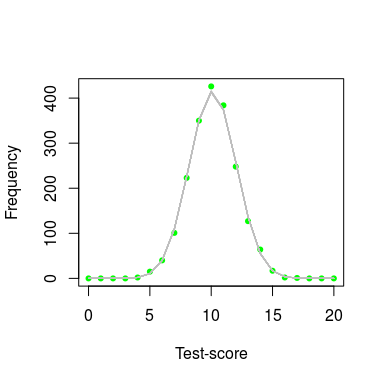The gray band is now not a single line but 20 lines superimposed, each of them showing the frequencies predicted from one of 20 draws from the posterior of the item parameters. As before, the green dots show the observed frequencies.

dbDisconnect(db2)